Home > CCA2 > Chapter 10 > Lesson 10.3.2 > Problem10-171

10-171.
1. Use the Binomial Theorem to write the expansion of each of the following expressions. Homework Help ✎

1.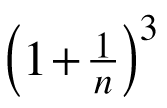2.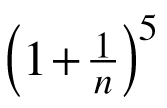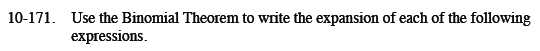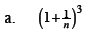$1+\frac{3}{n}+\frac{3}{n^2}+\frac{1}{n^3}$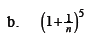Use the same method as in part (a).